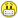Surprisingly enough, zero is an extremely important number used all the time in modern mathematics. However, the concept of zero was not that obvious in the past. The ancient Greeks could somehow do without a zero, even though they had already managed to create a very solid foundation for advanced geometry. The Romans, on the other hand, were happy enough using specific symbols which are today referred to as Roman numerals, like M, D, C, L, X, V and I. It is hard to believe that zero as a number had not been known in Europe until the late Middle Ages.

Only at the beginning of the 13th century an Italian mathematician, Leonardo Fibonacci of Pisa, dared to introduce the number zero in his historic mathematical treatise "Liber abaci". Sure enough, he must have been familiar with the idea of zero having traveled and studied in Africa and the Middle East where he was instructed by great Arab and Indian mathematicians of the time. Interestingly, he was the one who introduced Arabic numerals as a critical basis for the modern decimal numeral system. In addition to that, he invented a pretty fancy number sequence later named for him the Fibonacci sequence.

These days we know it well that zero is a regular number. No doubt about it. It is good to remember, however, that zero is also used as a positional digit in the decimal notation system playing the role of a placeholder which helps us easily discriminate between such numbers as 35 od 305, 3005 or 350. Some people claim that zero is neither an even nor an odd number. Let us remember that an even number is defined as the one divisible by two with no remainder. Number zero kindly complies with the definition since 0 : 2 = 0 (remainder equals 0) which is to be expected, if you think of it, because there can be no remainder left where there was nothing in the first place. Let us then remember that zero is an even number!!!

 !!! ZERO IS AN EVEN NUMBER !!!

Inrestingly, there is a whole section of mathematics dealing with the concept of a remainder and its calculation called modular arithmetic. In computer science the calculation of a remainder is trivial enough thanks to the modulo operator denoted by the percentage symbol % . It is one of the reasons why I love computer programming. It can do a lot of hard work for you in no time!It is just enough to type in the Perl or PHP code something like: \$remainder = 12894 % 33, and in a fraction of a second you will see that the variable \$remainder equals 24. In other words, as we would put it down during math class: 12894 : 33 = 390 remainder 24.

I have made a remainder calculator module for I believe that it might be useful to double-check stuff doing our math homework assignment someday!Tricky arithmetic involving zero

Let's assume that x is a variable whose value can change, i.e. it can store any arbitrary number. Hopefully, the equations presented below are kind of obvious:

x + 0 = x (addition)

0 + x = x (addition is commutative)

x − 0 = x (subtraction)

0 − x = −x (subtraction is NOT commutative!)

x • 0 = 0 (multiplication)

0 • x = 0 (multiplication is commutative)

x = 0 (zero raised to the power of x, where x > 0)

Powering with zero as an exponent seems less obvious:

x 0 = 1 (x to the power of 0, where x ≠ 0 !)

0 0 = ?  is mostly interpreted as an undefined expression,

however according to the combinatorial interpretation 0 0 = 1 !

And now a real challenge. How much is:

x : 0 = ? (division by zero!)

A question mark finishing off the above equation is absolutely justified as nobody in the whole world knows what the result of dividing any number by zero is. If you try it with your trusty calculator it will yell "ERROR!" at you in any given language or will simply report an "undefined value".As they jokingly say, black holes in outer space whose nature is still pretty much a mystery to us, were formed when God tried to divide a number by zeroWell, if you try to equally divide an empty bag of candy between x friends (assuming that the number of your friends > 0) it will, not surprisingly at all, give them zero satisfaction for:

0 : x  = 0 (where x ≠ 0 !)

Resuming, let us remember once and for all that dividing any number by 0 (including zero itself!) is illegal!!!

: 0 = ILLEGAL OPERATION !!!

0 : 0 = ILLEGAL OPERATION (INDETERMINATE FORM) !!!

 !!! DIVISION BY ZERO IS ILLEGAL !!!

Funnily, there are some very unexpected expressions one may see or hear in certain sports disciplines pertaining to the null result or number of points. In table tennis, for that matter, as well as in other sports involving bats and rackets, zero points is referred to as loveLegend has it that the term derives from the French word l'oeuf meaning "an egg" because of the characteristic shape of the digit zero. However, l'oeuf and love are pronounced in a substantially different manner, and besides, the French simply use the word zéro in this context which doesn't make the egg hypothesis very plausible.